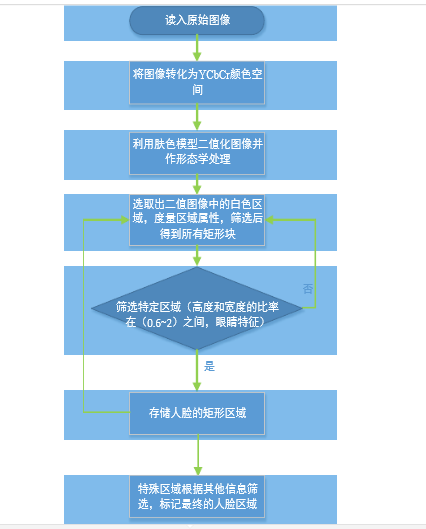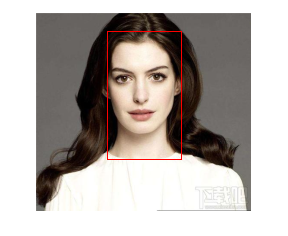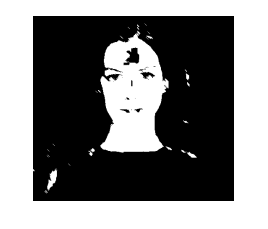﻿ 16074307 汪丽琼 数学建模实训 作业3 - 计算数学达人 - 专，学者，数值代数，微分方程数值解

### 16074307 汪丽琼 数学建模实训 作业3

1基本原理

2 算法思路

3 执行流程4 图片运行结果5  作业代码

function result = skin(Y,Cb,Cr)

%SKIN Summary of this function goes here

%Detailed explanation goes here

a=25.39;

b=14.03;

ecx=1.60;

ecy=2.41;

sita=2.53;

cx=109.38

cy=152.02;

xishu=[cos(sita) sin(sita);-sin(sita) cos(sita)];

%如果亮度大于230，则将长短轴同时扩大为原来的1.1

if(Y>230)

a=1.1*a;

b=1.1*b;

end

%根据公式进行计算

Cb=double(Cb);

Cr=double(Cr);

t=[(Cb-cx);(Cr-cy)];

temp=xishu*t;

value=(temp(1)-ecx)^2/a^2+(temp(2)-ecy)^2/b^2;

%大于1则不是肤色，返回0；否则为肤色，返回1

if value>1

result=0;

else

result=1;

end

end

function eye = findeye(bImage,x,y,w,h)

%FINDEYE Summary of this function goes here

%   Detailed explanation goes here part=zeros(h,w); %二值化

for i=y:(y+h)

for j=x:(x+w)

if bImage(i,j)==0

part(i-y+1,j-x+1)=255;

else

part(i-y+1,j-x+1)=0;

end

end

end

[L,num]=bwlabel(part,8);

%如果区域中有两个以上的矩形则认为有眼睛

if num<2

eye=0;

else

eye=1;

end

end

clear all;

%读入原始图像

gray=rgb2gray(I);

ycbcr=rgb2ycbcr(I);%将图像转化为YCbCr空间

heighth=size(gray,1);%读取图像尺寸

width=size(gray,2);

for i=1:heighth %利用肤色模型二值化图像

for j=1:width

Y=ycbcr(i,j,1);

Cb=ycbcr(i,j,2);

Cr=ycbcr(i,j,3);

if(Y<80)

gray(i,j)=0;

else

if(skin(Y,Cb,Cr)==1)%根据色彩模型进行图像二值化

gray(i,j)=255;

else

gray(i,j)=0;

end

end

end

end

se=strel('arbitrary',eye(5));%二值图像形态学处理

gray=imopen(gray,se);

figure;imshow(gray)

[L,num]=bwlabel(gray,8);%采用标记方法选出图中的白色区域

stats=regionprops(L,'BoundingBox');%度量区域属性

n=1;%存放经过筛选以后得到的所有矩形块

result=zeros(n,4);

figure,imshow(I);

hold on;

for i=1:num %开始筛选特定区域

box=stats(i).BoundingBox;

x=box(1);%矩形坐标X

y=box(2);%矩形坐标Y

w=box(3);%矩形宽度w

h=box(4);%矩形高度h

ratio=h/w;%宽度和高度的比例

ux=uint16(x);

uy=uint8(y);

if ux>1

ux=ux-1;

end

if uy>1

uy=uy-1

; end

if w<20 || h<20|| w*h<400 %矩形长宽的范围和矩形的面积可自行设定

continue

elseif ratio<2 && ratio>0.6 && findeye(gray,ux,uy,w,h)==1

%根据三庭五眼规则高度和宽度比例应该在（0.6,2）内；

result(n,:)=[ux uy w h];

n=n+1;

end

end
if size(result,1)==1 && result(1,1)>0 %

rectangle('Position',[result(1,1),result(1,2),result(1,3),result(1,4)],'EdgeColor','r');

else

%如果满足条件的矩形区域大于1,则再根据其他信息进行筛选

a=0;

arr1=[];arr2=[];

for m=1:size(result,1)

m1=result(m,1);

m2=result(m,2);

m3=result(m,3);